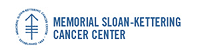Memorial Sloan-Kettering Cancer Center, Dept. of Epidemiology & Biostatistics Working Paper Series

Abstract

We consider the problem of selecting an optimal cutpoint for a continuous marker when the outcome of interest is subject to right censoring. Maximal chi square methods and receiver operating characteristic (ROC) curves-based methods are commonly-used when the outcome is binary. In this article we show that selecting the cutpoint that maximizes the concordance, a metric similar to the area under an ROC curve, is equivalent to maximizing the Youden index, a popular criterion when the ROC curve is used to choose a threshold. We use this as a basis for proposing maximal concordance as a metric to use with censored endpoints. Through simulations we evaluate the performance of two concordance estimates and three chi-square statistics under various assumptions. Maximizing the partial likelihood ratio test statistic has the best performance in our simulations.

Disciplines

Biostatistics | Statistical Models | Survival Analysis

COinS